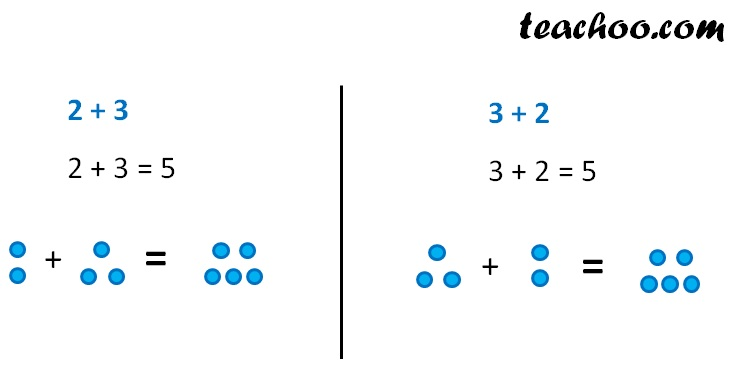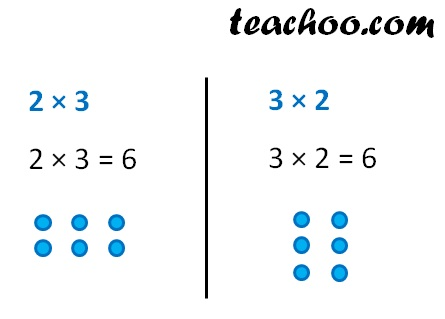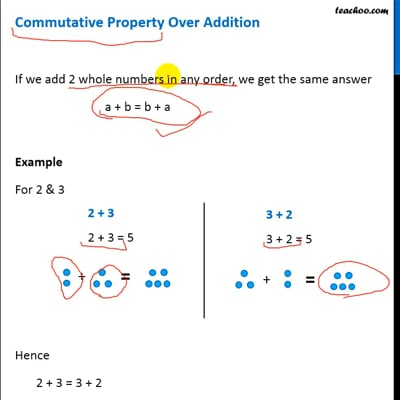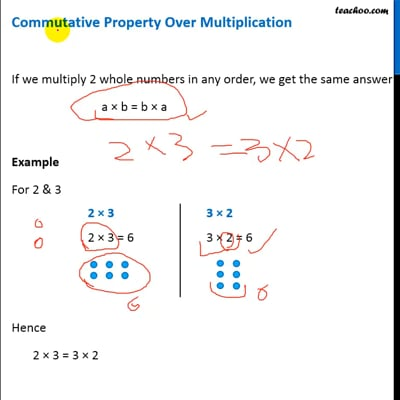Properties of whole numbers

Chapter 2 Class 6 Whole Numbers
Concept wise

If we add 2 whole numbers in any order, we get the same answer

a + b = b + a

Example

For 2 & 3Hence

2 + 3 = 3 + 2

### Commutative Property Over Multiplication

If we multiply 2 whole numbers in any order, we get the same answer

a × b = b × a

Example

For 2 & 3Hence

2 × 3 = 3 × 2This video is only available for Teachoo black usersThis video is only available for Teachoo black users

Introducing your new favourite teacher - Teachoo Black, at only ₹83 per month

### Transcript

Commutative Property Over Addition If we add 2 whole numbers in any order, we get the same answer a + b = b + a Example For 2 & 3 Hence 2 + 3 = 3 + 2 Commutative Property Over Multiplication If we multiply 2 whole numbers in any order, we get the same answer a × b = b × a Example For 2 & 3 Hence 2 × 3 = 3 × 2